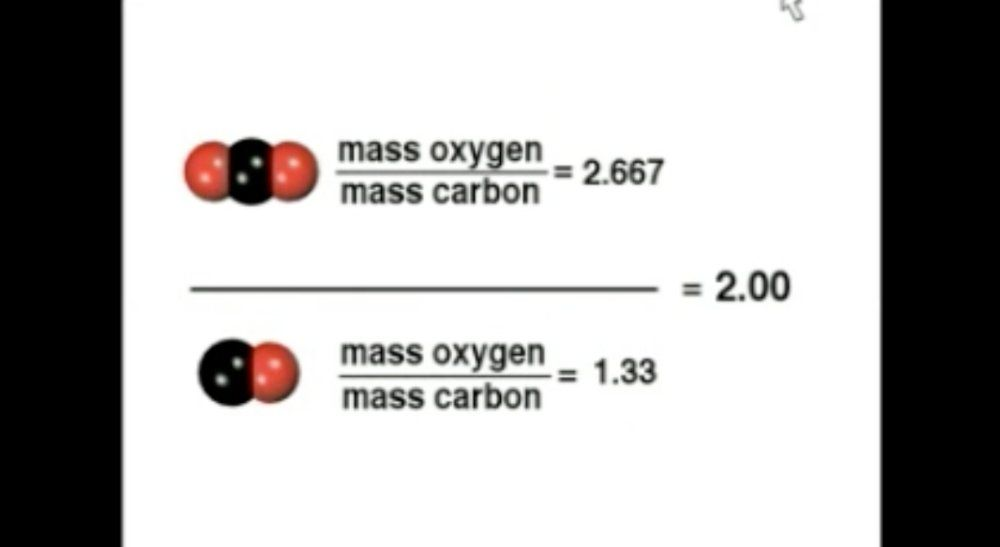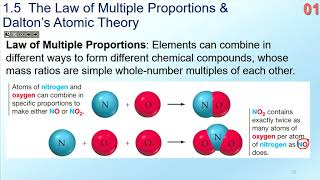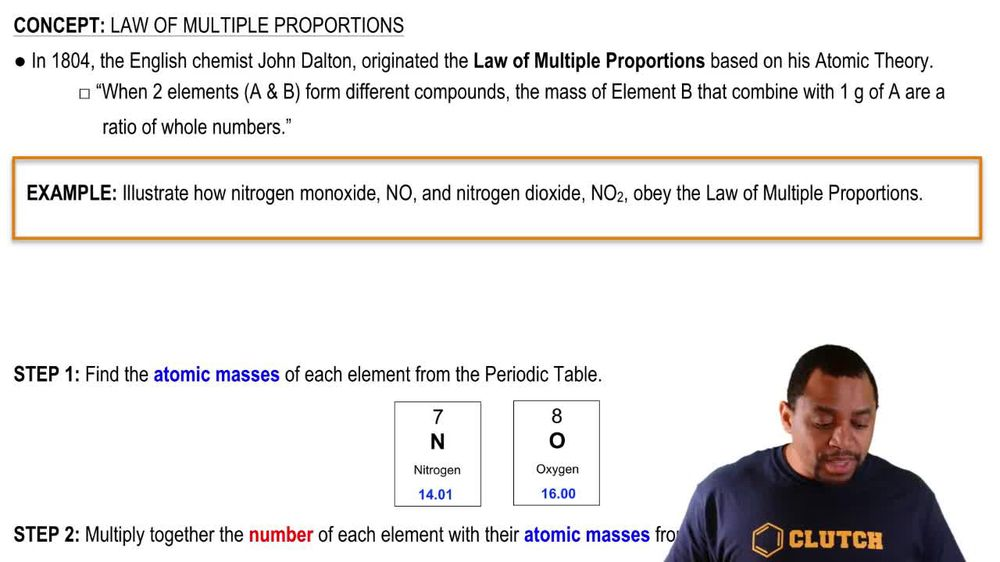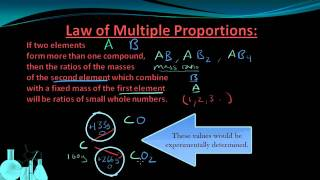Start typing, then use the up and down arrows to select an option from the list.
1. 2. Atoms & Elements2. Law of Multiple Proportions
Problem

# The ratio of sulfur to oxygen by mass in SO2 is 1.0:1.0. a. Find the ratio of sulfur to oxygen by mass in SO3. b. Find the ratio of sulfur to oxygen by mass in S2O.

Relevant Solution2m
Play a video:
Hey everyone in this example, we're told that samples of two different nitrogen and oxygen containing compounds were analyzed and decomposed into the elements. The first sample yielded 1.59 g of nitrogen and 0.91 g of oxygen, whereas the second sample yielded 0.76 g of nitrogen and 1.74 g of oxygen. We need to calculate the oxygen to nitrogen mass ratio for the two compounds. So we want to recall that our mass ratio is going to equal in our numerator, we're going to have our smaller mass, which is going to be our mass of oxygen in this case divided by our larger mass, which is given in the prompt as the mass of nitrogen. and so for our sample one We want to find that mass ratio. So what we're going to take is in our numerator, our mass that was smaller, which is given as .91 g of oxygen. And in our denominator we're going to have our mass of nitrogen given in the pram for sample one as 1.59 g of nitrogen. And this gives us a value of 0.572 as our mass ratio for sample one. Moving on to sample to We're gonna have a mass ratio in our numerator where we're plugging in our massive oxygen given in the prompt for sample to as 1.74 g. And then in our denominator we're gonna have our massive nitrogen Given as 0.76 g for our second sample. And so this gives us our mass ratio of oxygen to nitrogen of 2. and so to complete this example, this would be our mass ratio for sample one of oxygen to nitrogen And sample to mass ratio of oxygen to nitrogen is the second calculated value 2.29. And this will complete this example as our final answer. I hope that everything I ran through is clear. But if you have any questions, please leave them down below and I will see everyone in the next practice video.